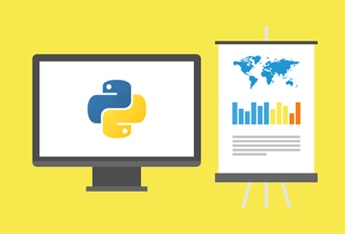## Course Details

Course Code: CP0101EN

Course Level: Beginner

Time to Complete: 3 hours

Language: English

Mathematical Programming is a powerful technique used to model and solve optimization problems. This training provides necessary fundamentals of mathematical programming and useful tips for good modelling practices to construct simple optimization models.

In this course, you will explore several aspects of mathematical programming to start learning more about constructing optimization models using IBM Decision Optimization technology, including:

• Basic terminology: operations research, mathematical optimization, and mathematical programming
• Basic elements of optimization models: data, decision variables, objective functions, and constraints
• Different types of solution: feasible, optimal, infeasible, and unbounded
• Mathematical programming techniques for optimization: linear programming, integer programming, mixed integer programming, and quadratic programming
• Algorithms used for solving continuous linear programming problems: simplex, dual simplex, and barrier
• Important mathematical programming concepts: sparsity, uncertainty, periodicity, network structure, convexity, piecewise linear and nonlinear
• These concepts are illustrated through concrete examples, including a production problem and different network models.

#### Course Syllabus

• What is Operations Research?
• What is Optimization?
• Optimization models
• Introduction to Linear programming
• A production problem : Part 1 - Writing the model
• A production problem : Part 2 - Finding a solution
• A production problem : Part 3 - From feasibility to unboundedness
• Algorithms for solving linear programs : Part 1 - The Simplex and Dual Simplex Algorithm
• Algorithms for solving linear programs : Part 2 - The Simplex and Barrier methods
• Introduction to Network Models
• The Transportation problem
• The Transshipment problem
• The Assignment problem
• The shortest path problem
• Critical path analysis
• Nonlinearity and Convexity
• Piecewise linear programming
• Integer programming
• The branch and bound method
• Modelling the real world
• The importance of Sparsity
• Tips for better models

#### General Information

• Self-paced
• Flexible Enrolment
• Audit multiple times

• None

• None

#### Course Staff#### Victoria Genin

Victoria Genin is an Information Developer at IBM in France. She works on IBM Decision Optimization products, writing documentation and creating video tutorials. She has a Master’s in Multimedia Content Creation from Paris Diderot University.#### Shirley de Jonk

Shirley de Jonk, PhD is a former UK University lecturer in Information Systems and researcher in Mathematical Programming. Today she is an Information Developer at IBM France and works on IBM Decision Optimization products.